# 傅里叶变换

### 概述

Fourier transformTransformée de Fourier有多个中文译名，常见的有“傅里叶变换”、“付立叶变换”、“傅立叶转换”、“傅氏转换”、“傅氏变换”、等等。

• 傅立叶变换是一种分析信号的方法，它可分析信号的成分，也可用这些成分合成信号。所谓信号，从狭义上说可以认为是自然界中作为信息载体的各类波，一般来说简谐震动产生的正弦波是最常见的研究对象。许多波形可作为信号的成分，比如正弦波、方波、锯齿波等，傅立叶变换用正弦波作为信号的成分。
• 对于自然界存在的所有波，我们可以利用所谓的傅立叶级数展开法将它们分解为有限或无限个不同频率不同振幅的正弦、余弦波的集合

### 定义

#### 连续傅里叶变换

• $f(t)$是$t$的周期函数，如果$t$满足狄里赫莱条件：在一个以 $2T$ 为周期内$f(X)$连续或只有有限个第一类间断点，附$f(x)$单调或可划分成有限个单调区间，则$F(x)$以$2T$为周期的傅里叶级数收敛，和函数$S(x)$也是以$2T$为周期的周期函数，且在这些间断点上，函数是有限值；在一个周期内具有有限个极值点；绝对可积。
• 则有下式成立，称为积分运算$f(t)$的傅立叶变换:

$$F(\omega)=\mathcal{F}[f(t)]=\int_{-\infty}^{\infty} f(t) e^{-i w t} d t$$

$F(\omega)$ 叫做 ${f}(\mathrm{t})$ 的象函数， ${f}({t})$ 叫做${F}(\omega)$ 的象原函数。

$F (\omega)$ 是 ${f}(\mathrm{t})$ 的象， $\mathrm{f}({t})$ 是$F(\omega)$原象。

• 傅里叶变换可以通过逆变换将象函数变换为象原函数

$$f(t)=\mathcal{F}^{-1}[F(\omega)]=\frac{1}{2 \pi} \int_{-\infty}^{\infty} F(\omega) e^{i w t} d \omega$$

• 傅立叶逆变换

$$f(t)=\mathcal{F}^{-1}[F(\omega)]=\frac{1}{2 \pi} \int_{-\infty}^{\infty} F(\omega) e^{i w t} d \omega$$

#### 离散傅里叶变换

• 设 $x(n)$ 是一个长度为 $M$ 的有限长序列，则定义 $x(n)$ 的 $N$ 点离散傅里叶变换为

$$X(k)=\operatorname{DFT}[x(n)]=\sum_{n=0}^{N-1} x(n) W_{N}^{k n} \quad k=0,1, \cdots, N-1$$

• $X(k)$ 的离散傅里叶逆变换(Inverse Discrete Fourier Transform, IDFT)为

$$x(n)=\operatorname{IDFT}[X(k)]=\frac{1}{N} \sum_{k=0}^{N-1} X(k) W_{N}^{-k n} \quad n=0,1, \cdots, N-1$$

### 相关概念

#### 时域频域的关系

• 傅里叶正变换和反变换，就是将信号在二者之间变换。
• 不同频率的正弦波相互正交，构成了频域空间上的基
• 傅里叶正变换就是当前的信号与所有频率不同相位的正弦信号计算点积，得到各个频率波上的分量，叠加构成当前的时域信号

### 实际应用

• 给出一幅图像，我们求出图像中圆形的周期和相位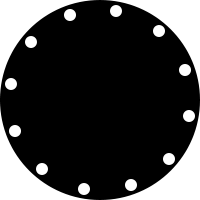• 将图像极坐标变换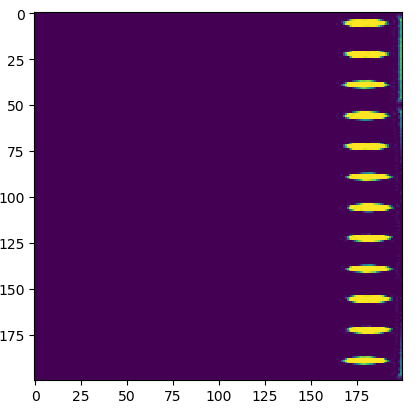• 叠加减去均值得到时域信号：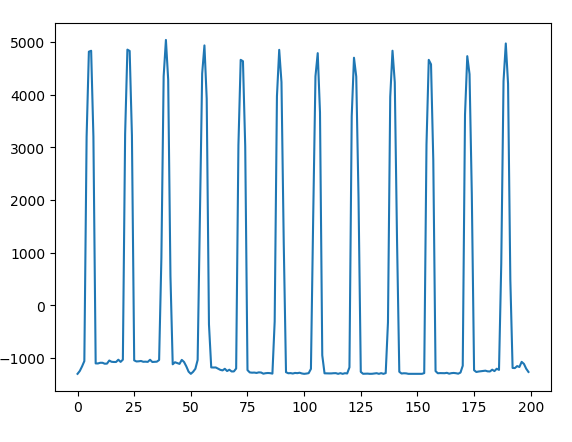• 离散傅里叶变换，计算模长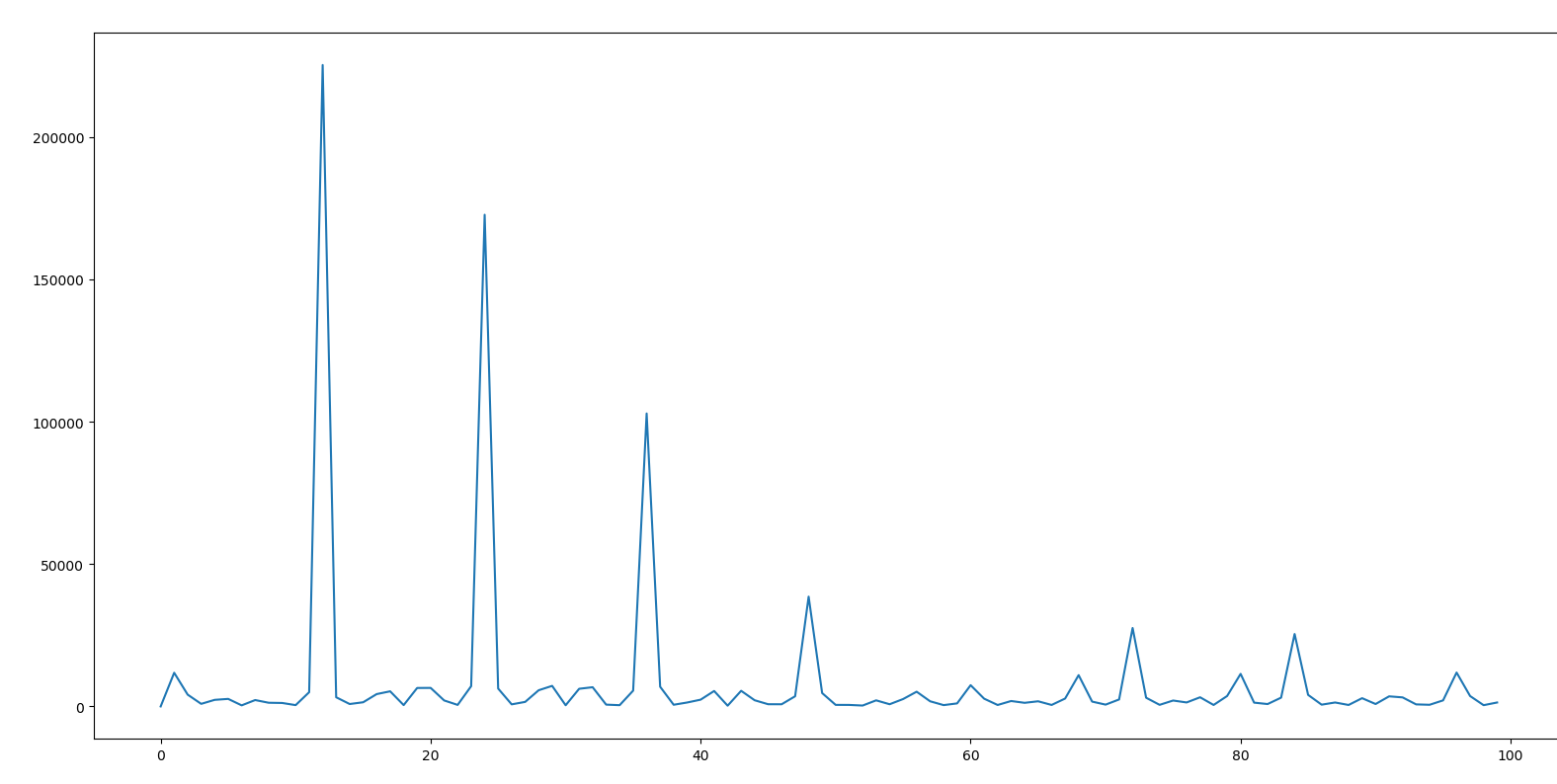• 其中能量最大的就是信号的周期 12，与实际相符

• 计算频率为12的相位，得到 -10.279644688897708，与实际相符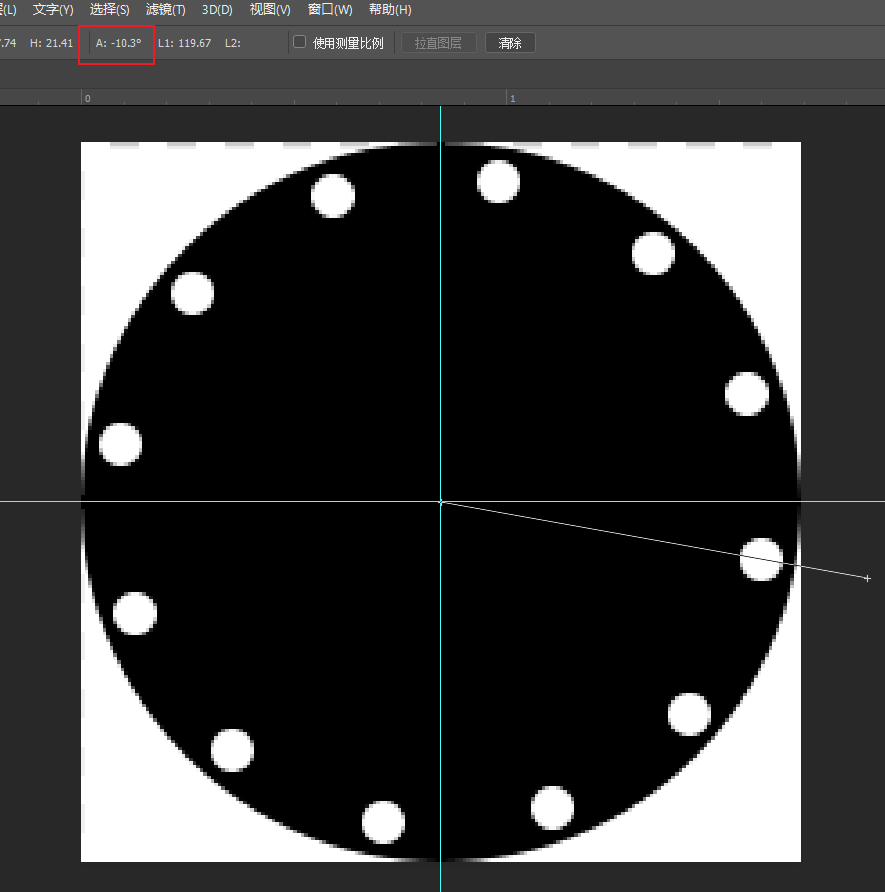### 参考资料

https://www.zywvvd.com/notes/study/math/fourier-transform/fourier-transform/

Yiwei Zhang

2021年5月29日